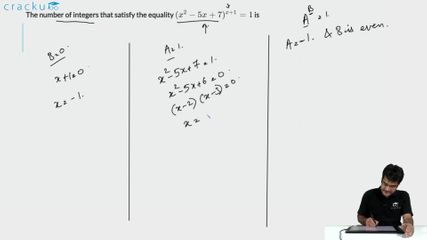Question 67

# The number of integers that satisfy the equality $$(x^{2}-5x+7)^{x+1}=1$$ is

Solution

$$\left(x^2-5x+7\right)^{x+1}=1$$

There can be a solution when $$\left(x^2-5x+7\right)=1$$ or $$x^2-5x\ +6=0$$

or x=3 and x=2

There can also be a solution when x+1 = 0 or x=-1

Hence three possible solutions exist.

### View Video Solution